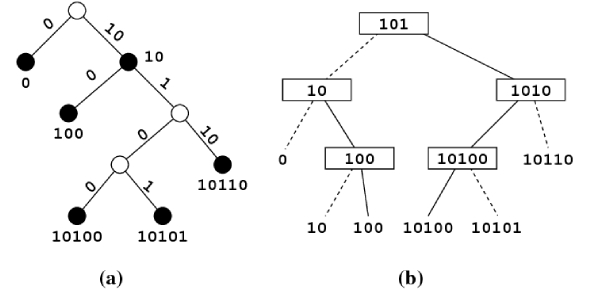# Toughest Data Structure Exam Quiz!

66 Questions | Total Attempts: 177SettingsCreate your own QuizData structure is the specific way to store and organize information so that the data can be successfully accessed. For this quiz, you should understand what a valid list operation is, the name of the second child vertex on a binary tree, all trees' property, how many children each vertex has, etc. This quiz will thoroughly prepare you for the most challenging data structure exam.

• 1.
Insert, Append, Delete, and Next are all valid list operations.
• A.

True

• B.

False

• 2.
Postfix notation may not contain negative numbers.
• A.

True

• B.

False

• 3.
An ordered tree in which each vertex has 0, 1, or 2 children.
• 4.
The name of the second child of a vertex on a binary tree.
• 5.
A node connected via edges to a higher node.
• 6.
If "X" is a descendent of "Y", then "Y" is a __________ of "X".
• 7.
All the descendents of a vertex.
• 8.
The length of the longest path from a vertex to a leaf that is a descendent of the vertex.
• A.

Height

• B.

Depth

• 9.
All parents have the same depth, but not necessarily the same height.
• A.

True - parents

• B.

False - siblings

• 10.
Property of all trees.
• A.

#Edges = #Nodes - 1

• B.

#Nodes = #Edges - 1

• 11.
An ordered tree in which each vertex has either no children, one child, or two children.
• A.

Sub Tree

• B.

Ordered Tree

• C.

Binary Tree

• 12.
Every vertex has two children or is a leaf.
• A.

Perfect Binary Tree

• B.

Full Binary Tree

• 13.
The "best case" search time for a B.S.T. is O(n). (where n = the number of nodes in the tree)
• A.

True

• B.

False

• 14.
A Red-Black tree is a BST tree.
• A.

True

• B.

False

• 15.
A BST tree is always balanced.
• A.

True

• B.

False

• 16.
All AVL trees are BST.
• A.

True

• B.

False

• 17.
For a normal (double-linked) binary tree, 8 pointers are assigned to each node.
• A.

True

• B.

False

• 18.
Inserting a node in an AVL tree will sometimes change the height of the tree.
• A.

True

• B.

False

• 19.
A hash table tends to perform better overall (bot time and space) than an array, linked list, or balanced B.S.T.
• A.

True

• B.

False

• 20.
Linear probing is not a good solution for clustering.
• A.

True

• B.

False

• 21.
Linear probing uses the number of times the rehash function has been applied as a value in the hash formula.
• A.

True

• B.

False

• 22.
What is the disadvantage of using buckets for collision resolution?
• A.

The search is long

• B.

It takes longer to walk down

• C.

Wasted space

• D.

Not knowing how big the bucket is

• E.

The return time

• 23.
Choose two ways to resolve problems following a deletion in a list using linear probing.
• A.

Use recursion

• B.

Using chain down the list

• C.

Shift Everything

• D.

Insert null in deleted vairable

• 24.
Reading a book (cover to cover) is an example of Pre Order Traversal.
• A.

True

• B.

False

• 25.
Which are Binary Trees?
• A.

A

• B.

B

• C.

C

• D.

D

• E.

E

Related TopicsBack to top
×

Wait!
Here's an interesting quiz for you.